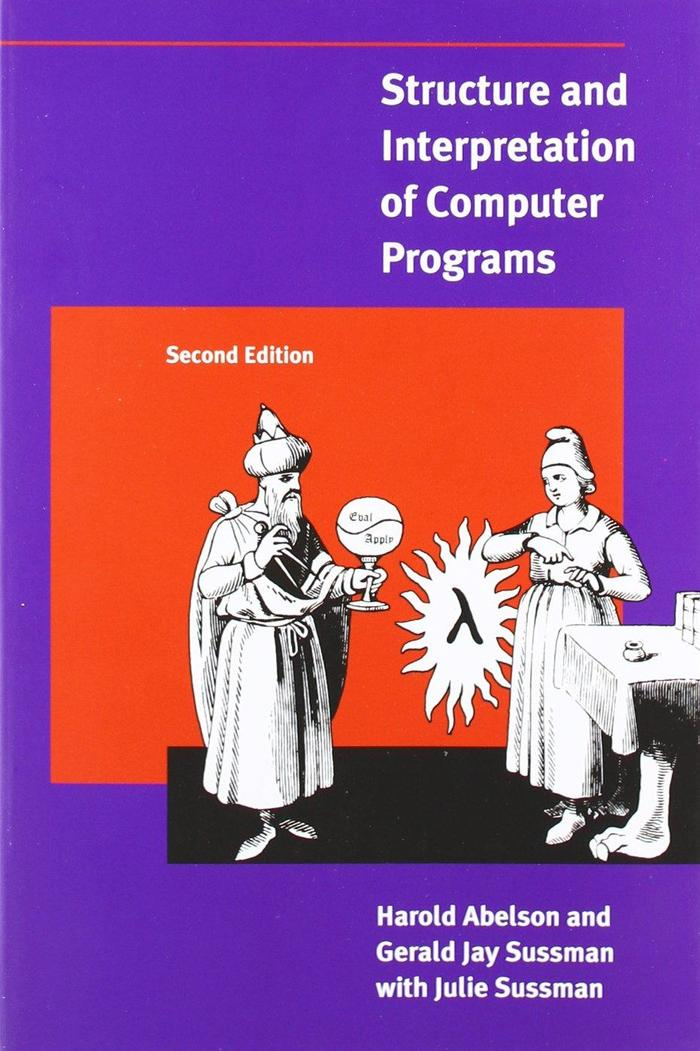18 Jun 2018

# A tour to Lisp

The Scheme dialect.At first, it seems that the only difference between lisp and other programing languages is the writing style: in Julia, it is

+(1, 2)


and in Scheme, a dialect of of Lisp,

(+ 1 2)


Other than the placement of brackets, there do not seem much difference. …Until, an exercise in sicp came up:

(define (a-plus-abs-b a b)
((if (> b 0) + -) a b))


Here, the operator + or - is determined by some condition. This clearly shows the benefit of the arrangement of brackets in Lisp: easily manipulate functions just as any data.

## Dive into julia-parser.scm

After the aperitif, let’s move to our real target: understand julia-parser.scm and modify it. The do-related function is,

(define (parse-do s)
(with-bindings
((expect-end-current-line (input-port-line (ts:port s))))
(without-whitespace-newline
(let ((doargs (if (memv (peek-token s) '(#\newline #\;))
'()
(parse-comma-separated s parse-range))))
(-> (tuple ,@doargs)
,(begin0 (parse-block s)
(expect-end s 'do)))))))


Some functions are used here:

### with-bindings

This function comes from the femtoLisp standard library.

(define-macro (with-bindings binds . body)
(let ((vars (map car binds))
(olds (map (lambda (x) (gensym)) binds)))
(let ,(map list olds vars)
,@(map (lambda (v val) (set! ,v ,val)) vars vals)
(unwind-protect
(begin ,@body)
(begin ,@(map (lambda (v old) (set! ,v ,old)) vars olds))))))


To understand it, we have to figure out what

• define-macro
• the  notation in (blabla)
• the , notation in ,(blabla) and ,v
• the ,@ notation in ,@(blabla)

the forms 'datum datum ,datum, and ,@datum denote two-element lists whose first elements are the symbols quote, quasiquote, unquote, and unquote-splicing, respectively. The second element in each case is datum.

### expect-end-current-line

This is a little bit strange. A number?

(define expect-end-current-line 0)


### input-port-line

It is a built-in function which receives a port and returns the line number. Note that port is the I/O stream for scheme.

### ts:port

Note: aref is the common-lisp name for vector-ref. i.e., get the $i$-th element of a vector.

        (define-macro (ts:port s) (aref ,s 1))


### without-whitespace-newline

; treat newline like ordinary whitespace instead of as a potential separator
(define whitespace-newline #f)

(define-macro (with-whitespace-newline . body)
(with-bindings ((whitespace-newline #t))
,@body))

(define-macro (without-whitespace-newline . body)
(with-bindings ((whitespace-newline #f))
,@body))


### memv

memv object list returns the first pair of list whose car is object. Returns #f if not found.

### #\newline

Characters are written using the notation #\character or #\character-name. For example: #\newline is the newline character.

### parse-comma-separated

(define (parse-comma-separated s what)
(let loop ((exprs '()))
(let ((r (what s)))
(case (peek-token s)
((#\,)
(take-token s)
(loop (cons r exprs)))
(else   (reverse! (cons r exprs)))))))


### parse-range

;; parse ranges and postfix ...
;; colon is strange; 3 arguments with 2 colons yields one call:
;; 1:2   => (call : 1 2)
;; 1:2:3 => (call : 1 2 3)
(define (parse-range s)
(let loop ((ex     (parse-expr s))
(first? #t))
(let* ((t   (peek-token s))
(spc (ts:space? s)))
(cond ((and first? (eq? t '|..|))
(take-token s)
(call ,t ,ex ,(parse-expr s)))
((and range-colon-enabled (eq? t ':))
(take-token s)
(if (and space-sensitive spc
(or (peek-token s) #t) (not (ts:space? s)))
;; "a :b" in space sensitive mode
(begin (ts:put-back! s ': spc)
ex)
(let ((argument
(cond ((closing-token? (peek-token s))
(error  (string "missing last argument in \""
(deparse ex) ":\" range expression ")))
((newline? (peek-token s))
(error "line break in \":\" expression"))
(else
(parse-expr s)))))
(if (and (not (ts:space? s))
(or (eq? argument '<) (eq? argument '>)))
(error (string "\":" argument "\" found instead of \""
argument ":\"")))
(if first?
(loop (list 'call t ex argument) #f)
(loop (append ex (list argument)) #t)))))
((eq? t '...)
(take-token s)
(list '... ex))
(else ex)))))
`